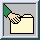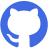### A Pseudorandom Number Sequence Test Program

This page describes a program, ent, which applies various tests to sequences of bytes stored in files and reports the results of those tests. The program is useful for evaluating pseudorandom number generators for encryption and statistical sampling applications, compression algorithms, and other applications where the information density of a file is of interest.

### NAME

ent - pseudorandom number sequence test

### SYNOPSIS

ent [ -b -c -f -t -u ] [ infile ]

### DESCRIPTION

ent performs a variety of tests on the stream of bytes in infile (or standard input if no infile is specified) and produces output as follows on the standard output stream:

```    Entropy = 7.980627 bits per character.

Optimum compression would reduce the size
of this 51768 character file by 0 percent.

Chi square distribution for 51768 samples is 1542.26, and randomly
would exceed this value less than 0.01 percent of the times.

Arithmetic mean value of data bytes is 125.93 (127.5 = random).
Monte Carlo value for Pi is 3.169834647 (error 0.90 percent).
Serial correlation coefficient is 0.004249 (totally uncorrelated = 0.0).
```

The values calculated are as follows:

Entropy
The information density of the contents of the file, expressed as a number of bits per character. The results above, which resulted from processing an image file compressed with JPEG, indicate that the file is extremely dense in information—essentially random. Hence, compression of the file is unlikely to reduce its size. By contrast, the C source code of the program has entropy of about 4.9 bits per character, indicating that optimal compression of the file would reduce its size by 38%. [Hamming, pp. 104–108]
Chi-square Test

The chi-square test is the most commonly used test for the randomness of data, and is extremely sensitive to errors in pseudorandom sequence generators. The chi-square distribution is calculated for the stream of bytes in the file and expressed as an absolute number and a percentage which indicates how frequently a truly random sequence would exceed the value calculated. We interpret the percentage as the degree to which the sequence tested is suspected of being non-random. If the percentage is greater than 99% or less than 1%, the sequence is almost certainly not random. If the percentage is between 99% and 95% or between 1% and 5%, the sequence is suspect. Percentages between 90% and 95% and 5% and 10% indicate the sequence is “almost suspect”. Note that our JPEG file, while very dense in information, is far from random as revealed by the chi-square test.

Applying this test to the output of various pseudorandom sequence generators is interesting. The low-order 8 bits returned by the standard Unix `rand()` function, for example, yields:

Chi square distribution for 500000 samples is 0.01, and randomly would exceed this value more than 99.99 percent of the times.

While an improved generator [Park & Miller] reports:

Chi square distribution for 500000 samples is 212.53, and randomly would exceed this value 97.53 percent of the times.

Thus, the standard Unix generator (or at least the low-order bytes it returns) is unacceptably non-random, while the improved generator is much better but still sufficiently non-random to cause concern for demanding applications. Contrast both of these software generators with the chi-square result of a genuine random sequence created by timing radioactive decay events.

Chi square distribution for 500000 samples is 249.51, and randomly would exceed this value 40.98 percent of the times.

See [Knuth, pp. 35–40] for more information on the chi-square test. An interactive chi-square calculator is available at this site.

Arithmetic Mean
This is simply the result of summing the all the bytes (bits if the -b option is specified) in the file and dividing by the file length. If the data are close to random, this should be about 127.5 (0.5 for -b option output). If the mean departs from this value, the values are consistently high or low.
Monte Carlo Value for Pi
Each successive sequence of six bytes is used as 24 bit X and Y co-ordinates within a square. If the distance of the randomly-generated point is less than the radius of a circle inscribed within the square, the six-byte sequence is considered a “hit”. The percentage of hits can be used to calculate the value of Pi. For very large streams (this approximation converges very slowly), the value will approach the correct value of Pi if the sequence is close to random. A 500000 byte file created by radioactive decay yielded:
Monte Carlo value for Pi is 3.143580574 (error 0.06 percent).
Serial Correlation Coefficient
This quantity measures the extent to which each byte in the file depends upon the previous byte. For random sequences, this value (which can be positive or negative) will, of course, be close to zero. A non-random byte stream such as a C program will yield a serial correlation coefficient on the order of 0.5. Wildly predictable data such as uncompressed bitmaps will exhibit serial correlation coefficients approaching 1. See [Knuth, pp. 64–65] for more details.

### OPTIONS

-b
The input is treated as a stream of bits rather than of 8-bit bytes. Statistics reported reflect the properties of the bitstream.
-c
Print a table of the number of occurrences of each possible byte (or bit, if the -b option is also specified) value, and the fraction of the overall file made up by that value. Printable characters in the ISO 8859-1 Latin-1 character set are shown along with their decimal byte values. In non-terse output mode, values with zero occurrences are not printed.
-f
Fold upper case letters to lower case before computing statistics. Folding is done based on the ISO 8859-1 Latin-1 character set, with accented letters correctly processed.
-t
Terse mode: output is written in Comma Separated Value (CSV) format, suitable for loading into a spreadsheet and easily read by any programming language. See Terse Mode Output Format below for additional details.
-u
Print how-to-call information.

### FILES

If no infile is specified, ent obtains its input from standard input. Output is always written to standard output.

### TERSE MODE OUTPUT FORMAT

Terse mode is selected by specifying the -t option on the command line. Terse mode output is written in Comma Separated Value (CSV) format, which can be directly loaded into most spreadsheet programs and is easily read by any programming language. Each record in the CSV file begins with a record type field, which identifies the content of the following fields. If the -c option is not specified, the terse mode output will consist of two records, as follows:

```0,File-bytes,Entropy,Chi-square,Mean,Monte-Carlo-Pi,Serial-Correlation
1,file_length,entropy,chi_square,mean,Pi_value,correlation
```

where the italicised values in the type 1 record are the numerical values for the quantities named in the type 0 column title record. If the -b option is specified, the second field of the type 0 record will be “File-bits”, and the file_length field in type 1 record will be given in bits instead of bytes. If the -c option is specified, additional records are appended to the terse mode output which contain the character counts:

```2,Value,Occurrences,Fraction
3,v,count,fraction
. . .
```

If the -b option is specified, only two type 3 records will appear for the two bit values v=0 and v=1. Otherwise, 256 type 3 records are included, one for each possible byte value. The second field of a type 3 record indicates how many bytes (or bits) of value v appear in the input, and fraction gives the decimal fraction of the file which has value v (which is equal to the count value of this record divided by the file_length field in the type 1 record).

### BUGS

Note that the “optimal compression” shown for the file is computed from the byte- or bit-stream entropy and thus reflects compressibility based on a reading frame of the chosen width (8-bit bytes or individual bits if the -b option is specified). Algorithms which use a larger reading frame, such as the Lempel-Ziv [Lempel & Ziv] algorithm, may achieve greater compression if the file contains repeated sequences of multiple bytes.

##Download random.zip (Zipped archive)

The program is provided as random.zip, a Zipped archive containing an ready-to-run Win32 command-line program, `ent.exe` (compiled using Microsoft Visual C++ .NET, creating a 32-bit Windows executable), and in source code form along with a `Makefile` to build the program under Unix.

###Source code repository at GitHub

Introduction to Probability and Statistics
[Hamming]
Hamming, Richard W. Coding and Information Theory. Englewood Cliffs NJ: Prentice-Hall, 1980. ISBN 978-0-13-139139-0.
[Knuth]
Knuth, Donald E. The Art of Computer Programming, Volume 2 / Seminumerical Algorithms. Reading MA: Addison-Wesley, 1969. ISBN 978-0-201-89684-8.
[Lempel & Ziv]
Ziv J. and A. Lempel. “A Universal Algorithm for Sequential Data Compression”. IEEE Transactions on Information Theory 23, 3, pp. 337-343.
[Park & Miller]
Park, Stephen K. and Keith W. Miller. “Random Number Generators: Good Ones Are Hard to Find”. Communications of the ACM, October 1988, p. 1192.

This software is in the public domain. Permission to use, copy, modify, and distribute this software and its documentation for any purpose and without fee is hereby granted, without any conditions or restrictions. This software is provided “as is” without express or implied warranty.